Published: 31 December 2016

# The 3D object recognition with environmental adaptability based on VFH descriptor and region growing segmentation

Zhuang Peng1
Jinbao Chen2
Dong Han3
Meng Chen4
1AVIC Chengdu Aircraft Industrial (Group) Co., Ltd., Chengdu, China
2, 3Nanjing University of Aeronautics and Astronautics, Nanjing, China
4Shanghai Institute of Aerospace Systems Engineering, Shanghai, China
Corresponding Author:
Zhuang Peng
Views 63

#### Abstract

3D object recognition is a basic research in the machine vision field. Microsoft KINECT V2 is utilized to collect external environmental information. The point cloud file is obtained after processing the collected information. In order to filter the point cloud and obtain point cloud model of a single object in the environment after region growing segmentation, the point cloud is applied to point cloud library. Then, the VFH descriptor of the point cloud model is calculated. After inputting point cloud model of the trained target, the point cloud model with the minimum CHI square distance between the VFH descriptor of the target and VFH descriptor of the point cloud model can be found. The 3D object corresponding to the found model is the identified object. For the 3D object recognition in an unfamiliar environment, the algorithm of 3D object recognition with environmental adaptability is proposed. After the 3D object recognition system built, the physical verification is conducted about the proposed algorithm. Giving the target model, the system successfully identifies the 3D object in the unfamiliar environment, that demonstrates the correctness of the algorithm.

## 1. Introduction

3D object recognition is so versatile, such as space exploration, industrial production and household services [1-4]. So, it is a priority that need to be solved. 3D object recognition is an artificial intelligence technology which mimic human to recognize and identify objects in the environment through the eyes. However, according to the fact that the human vision occupies at least 60 percent of the human brain resources, 3D object recognition is considered as AI-complete problems in academic circles .

3D object recognition is generally divided into three steps. Firstly, it trains the target object and calculates the feature vector of the target. Then, it segments various objects in the environment and calculates the feature vector of each object. Finally, it gets the feature vector closest to the target feature vector. And its corresponding object is the target. Y. Guo et al. used rotational projection statistics for 3D local surface description and object recognition . The type of data they used is MESH type which is more difficult to obtain. Steder et al. proposed NARF descriptors for object recognition . In order to make the descriptor invariant to rotation, they extracted a unique orientation from the descriptor and shifted the descriptor according to this orientation. G. Zhou et al. proposed a method which uses the salient points as the key point to recognize the object . But this method is limited for identifying an object with a smooth surface.

In order to identify the object in the unfamiliar environment, this paper proposes a method which adjusts the parameter dynamically so that the region growing segmentation can adapt to the environment. VFH descriptor is used to describe the object feature, and the target object is judged by CHI square distance of VFH descriptors. Finally, the research builds the 3D object recognition system. It successfully identifies the irregular objects, that verifies the correctness of the method.

## 2. Point cloud acquisition

This paper utilizes KINECT V2 to integrate depth information and color information into a three-dimensional point cloud with color components. Firstly, a KINECT class is initialized. Then it gets color image frame and depth image frame resources and obtains the coordinates mapper. After creating the viewer classes of those resources, the data of the latest frame are acquired. Integration of color and depth resources needs to use already acquired coordinate mapper, because of the difference between coordinate system origin of two resources. The coordinates of current depth point in the color image frame are obtained in the coordinate mapper. Then, RGB values of the current depth point are obtained. And it gets $XYZ$ values of the current depth point in the camera space coordinate system. The RGB values and the $XYZ$ values of the current depth point are assigned to a Point XYZRGB-type point of PCL. After the above operations are done for each the point in the current depth frame, the model of the convenient-interactive colorful point cloud is obtained (Fig. 1(a)). In the following, this paper will use the point cloud model without color information (Fig. 1(b)).

Fig. 1Point cloud model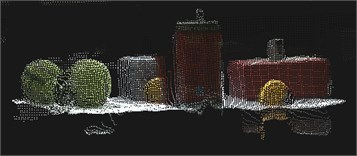a) With color information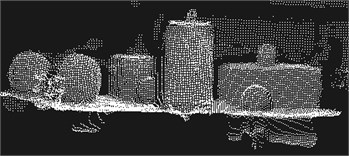b) Without color information

## 3. Region growing segmentation

Due to the instability of KINECT V2 sensor and the environmental factors, there are some noise in the model of raw point cloud (Fig. 1(b)). It may lead to calculation error of partial features of point cloud model, such as curvature and normal, thus resulting in the point cloud segmentation fail. In order to remove those points not meeting the criteria, statistical analysis for near point of each point is done . For each point, the mean distance from it to all its neighbors is calculated. By assuming that the resulted distribution is Normal Distribution with a mean and a standard deviation, those points whose mean distance is outside an interval (Eq. (1)) can be considered as outliers. The interval has following formula:

1
$I=\mu ±\sigma \cdot k,$

where $I$ represents the interval, $\mu$ is the mean and $\sigma$ is the standard deviation, $k$ is standard deviation coefficient.

The number of point’s neighbors is 50 and $k$ is 0.5 in this paper. After outliers trimmed from the raw point cloud model, the new point cloud model becomes more real (Fig. 2).

Fig. 2Filtered point cloud model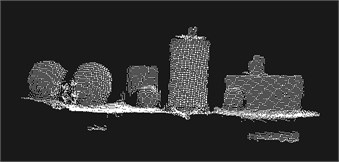Fig. 3Point cloud model after region growing segmentation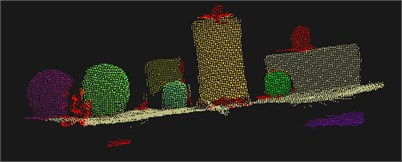Then, this paper implements region growing segmentation on filtered point cloud model . First of all, it computes the normal and curvature value of each point in point cloud model. It sorts the points by their curvature value. Region growth begins from the point having the smallest curvature value, because growth from the flattest area allows to reduce the total number of segments. The main process of region growth is as follows:

1) It picks up the point with minimum curvature value.

2) The picked point is added to the set called seeds.

3) For every seed point, the algorithm finds its neighbors.

4) Calculating the angle between the normal of seed point and the normal of each neighbor point, if the angle is less than threshold value, then current point is added to the current region.

5) For every neighbor, if the curvature is less than threshold value, this point is added to the seeds. And the current seed point is removed from the seeds.

Repeating the main process, if the seeds becomes empty, the growth of the picked point with minimum curvature value is completed. Removing those points of growth region from the model, it sorts the points by their curvature value again. Then it repeats the above-mentioned method. This paper sets the number of neighbors to 30, the angle threshold of normal to 0.05Π and curvature threshold to 40. Then it gets those regions after region growing segmentation (Fig. 3). There different colors regions represent different objects, and non-touching regions of the same color also represent different objects.

## 4. Compute VFH descriptor

VFH descriptor is a novel representation for object features . VFH descriptor consists of an extended FPFH of center point and the angle between the central viewpoint direction translated to each normal. Center point is the point with the minimum mean distance from itself to all points in the point cloud. The extended FPFH includes the relative pan, tilt, yaw angles and the distances between each point and the center point (Fig. 4).

Fig. 4The extended FPFH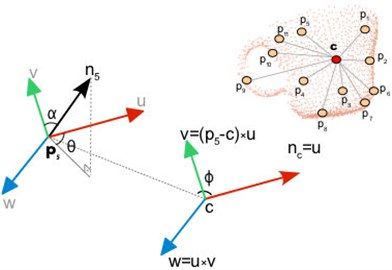Fig. 5Viewpoint direction angle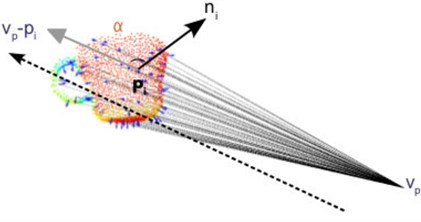Where $c$ is center point and ${n}_{c}$ is normal of $c$ and ${p}_{5}$ is a point in point cloud model and ${n}_{5}$ is normal of ${p}_{5}$. Defining Cartesian coordinate system, where $u$ is unit vector with the same direction of ${n}_{c}$ and $v$ is unit vector with the same direction of $\left({p}_{5}-c\right)×u$ and $w$ is unit vector with the same direction of $u×v$. So, there are the following formulas:

2
$\alpha =v\cdot {n}_{5},$
3
$\varphi =u\cdot \frac{{p}_{5}-c}{d},$
4
$\theta =\mathrm{a}\mathrm{r}\mathrm{c}\mathrm{t}\mathrm{a}\mathrm{n}\left(w\cdot {n}_{5},u\cdot {n}_{5}\right),$
5
$d={‖{p}_{5}-c‖}_{2}.$

$\alpha$, $\varphi$ and $\theta$ between center point and other points are computed, which is called the Simplified Point Feature Histogram (SPFH). Then:

6
$FPFH\left(c\right)=SPFH\left(c\right)+\frac{1}{k}{\sum }_{i=1}^{k}\frac{1}{{d}_{k}}\cdot SPFH\left({p}_{k}\right),$

where $k$ is the number of points in the sphere and the sphere’s center is $c$ and the sphere’s radius is the distance from $c$ to the farthest point and ${d}_{k}$ is the distance from $c$ to ${p}_{k}$.

Then, the angle between the central viewpoint direction translated (black dotted line in Fig. 5, not gray line) to each normal is computed by SPFH method (Fig. 5).

The VFH uses 45 binning subdivisions for each of the four extended FPFH values and 128 binning subdivisions for the viewpoint component. There are the VFH descriptors (Fig. 6) of the yellow cylinder region and the biggest blue sphere region in the Fig. 3.

Comparing the two VFH descriptors in Fig. 6, it can demonstrate that VFH descriptors of different shape and pose objects are different. So, this paper will take advantage of this feature for 3D object recognition.

Fig. 6VFH descriptors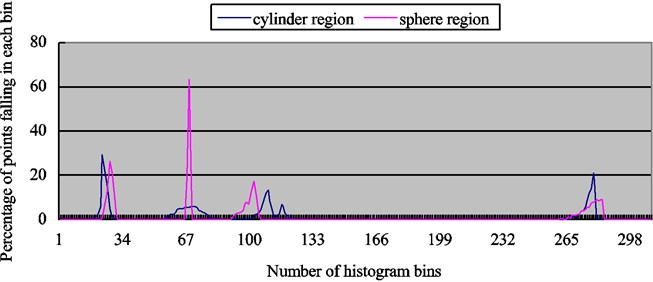## 5. 3D object recognition and the algorithm with environmental adaptability

3D object recognition process is as follows:

1) Establish point cloud model of the target object and calculate its VFH descriptor.

2) Establish point cloud model of the environment.

3) Filter point cloud model of the environment and complete region growing segmentation.

4) Calculate VFH descriptor of each region.

5) Calculate CHI square distance between target’s VFH descriptor and each region’s.

6) Find out the region with minimum CHI square distance that is the target object.

In fact, the algorithm has some faultiness that there are two objects not segmented correctly in Fig. 3. It must improve the algorithm for 3D object recognition in the unfamiliar environment. So, the algorithm of 3D object recognition with environmental adaptability is proposed. Aiming the minimum CHI square distance, the algorithm adjusts parameters to make 3D object recognition system adapt to the unfamiliar environment. According to the characteristics of the algorithm, the parameters of VFH descriptor calculation should be prespecified. After testing, the curvature threshold value is set to 40 that has a small impact on the region growing segmentation. So, the angle threshold of normal could be only adjusted dynamically.

It calculates CHI square distance between VFH descriptors of target and each region after each segmentation, where angle threshold of normal decreases from 30 degrees to 1 degree (Step: 1 degree). So, the region with minimum CHI square distance in 30 times segmentation can be found and its corresponding threshold is the parameter best adapted to the current environment. And the region is identified as the target object.

## 6. Experimental verification

There is the point cloud model of the unfamiliar environment in Fig. 7(a) and the target object model in Fig. 7(c) which has been established previously. The best angle threshold of normal is 16 degrees in this unfamiliar environment after algorithm is executed. There are regions after region growing segmentation in Fig. 7(b) and the recognition result in Fig. 7(d).

Fig. 7Point cloud model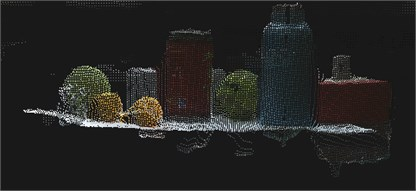a) The unfamiliar environment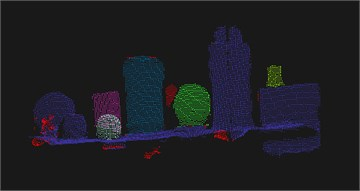b) The regions after region growing segmentation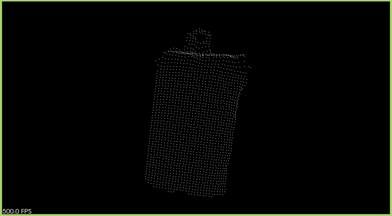c) The target object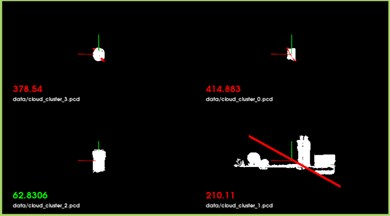d) The recognition result

Fig. 8Point cloud model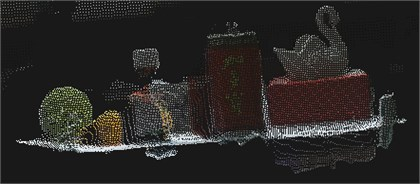a) The unfamiliar environment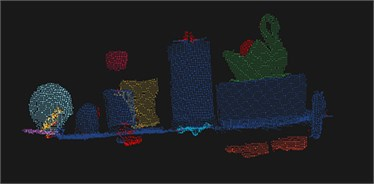b) The regions after region growing segmentation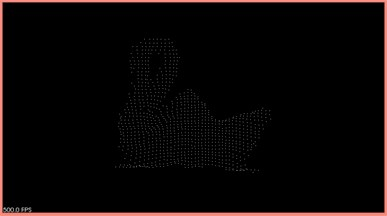c) The target object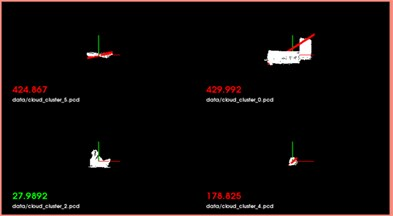d) The recognition result

The identified object is the region of the lower left corner in Fig. 7(d) which is corresponding to the red cylinder object in the environment. Where 62.8306 is CHI square distance between the VFH descriptors of the target and actual object. The region of the lower right corner is not divided and includes 5 objects. If it includes the target, the angle threshold of normal will be adjusted adaptively. Obviously, the relationship between the angle threshold of normal and the CHI square distance is nonlinear. Once the target has been segmented, it will be divided out in the next division, or divided into some smaller regions. That is why angle threshold of normal decreases from 30 degrees to 1 degree (Step: 1 degree).

Next, this paper verifies an irregular object recognition. There is the point cloud model of the unfamiliar environment in Fig. 7(a) and the target object model in Fig. 7(c) which has been established previously. The best angle threshold of normal is 14 degrees in this unfamiliar environment after algorithm is executed. There are regions after the region growing segmentation in Fig. 7(b) and the recognition result in Fig. 7(d).

## 7. Conclusions

Firstly, this paper builds point cloud model and segments the model by region growing segmentation. Then, it calculates the VFH descriptor of each region. The algorithm of 3D object recognition with environmental adaptability is proposed. The algorithm can make 3D object recognition system adapt to the unfamiliar environment and segment each object correctly. By the minimum CHI square distance of the VFH descriptors standards, it determines the angle threshold of normal. Finally, the system completes the 3D object recognition accurately, including regular object and irregular object.

However, it finds that the direction of the viewpoint has a significant impact on the object recognition. So, establishing the point cloud model of the multi-viewpoint direction of the target object is extremely important. In the future, further studies will be launched.

#### Cited by

2023 IEEE International Conference on Robotics and Automation (ICRA)